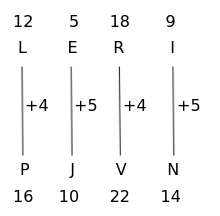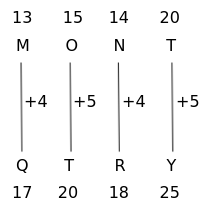Question 5

# In the following question, select the related letters from the given alternatives.LERI : PJVN :: MONT : ?

Solution

Given, LERI is related to PJVN

The logic here isSimilarly,$$\therefore\$$MONT is related to QTRY in the same way LERT is related to PJVN

Hence, the correct answer is Option B

• Free SSC Study Material - 18000 Questions
• 230+ SSC previous papers with solutions PDF